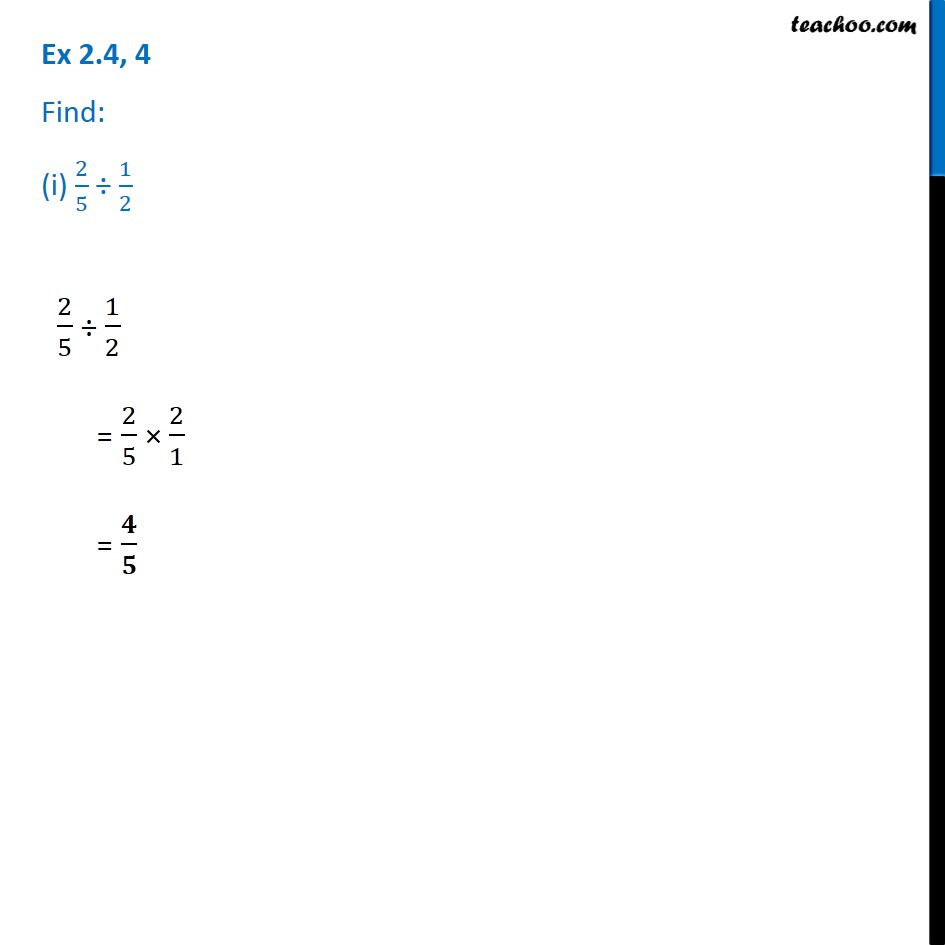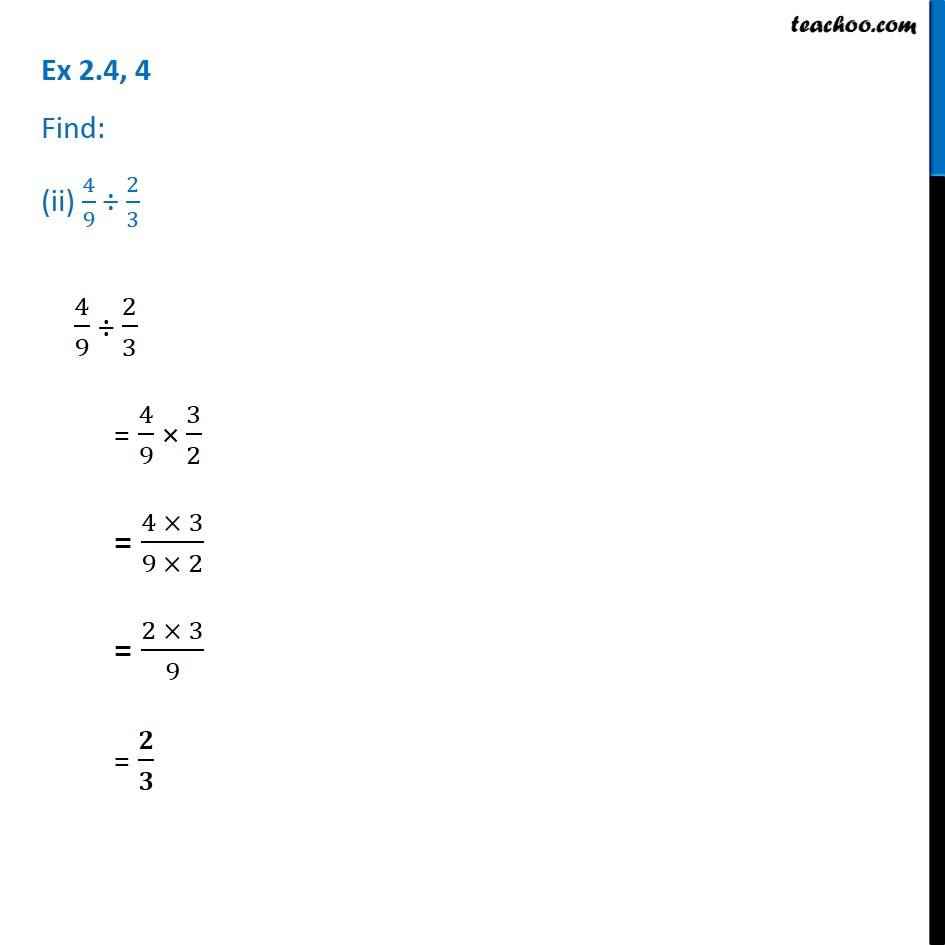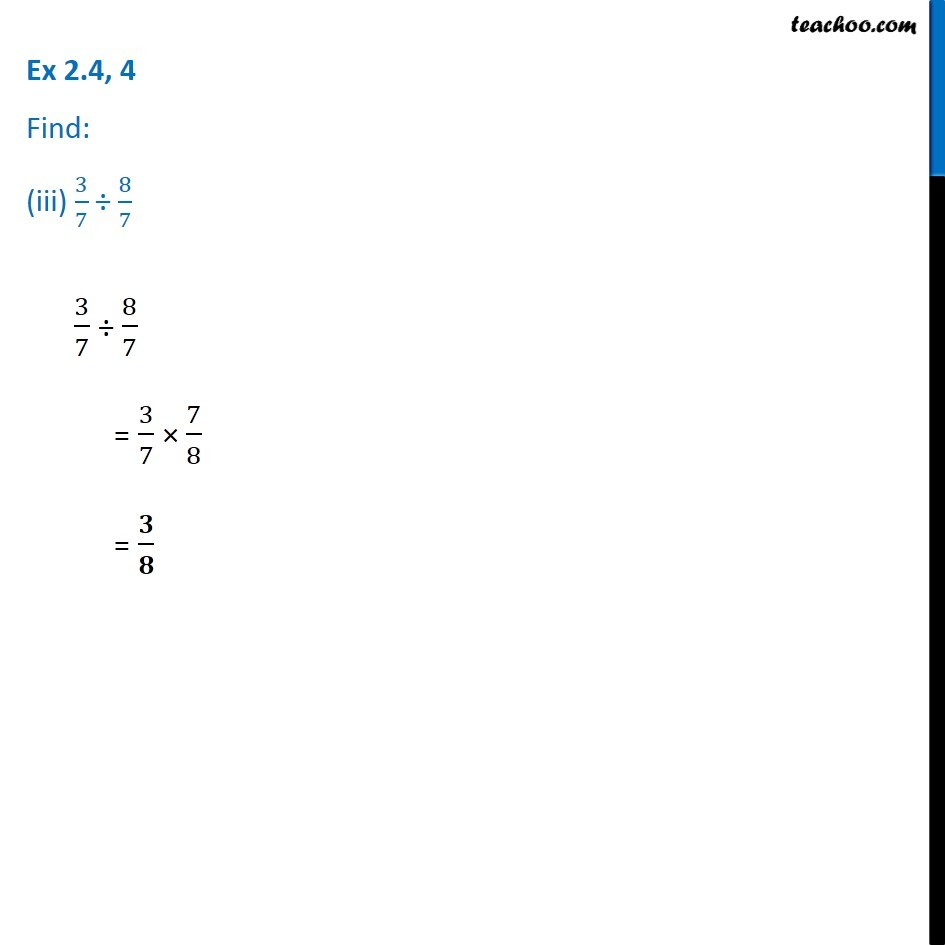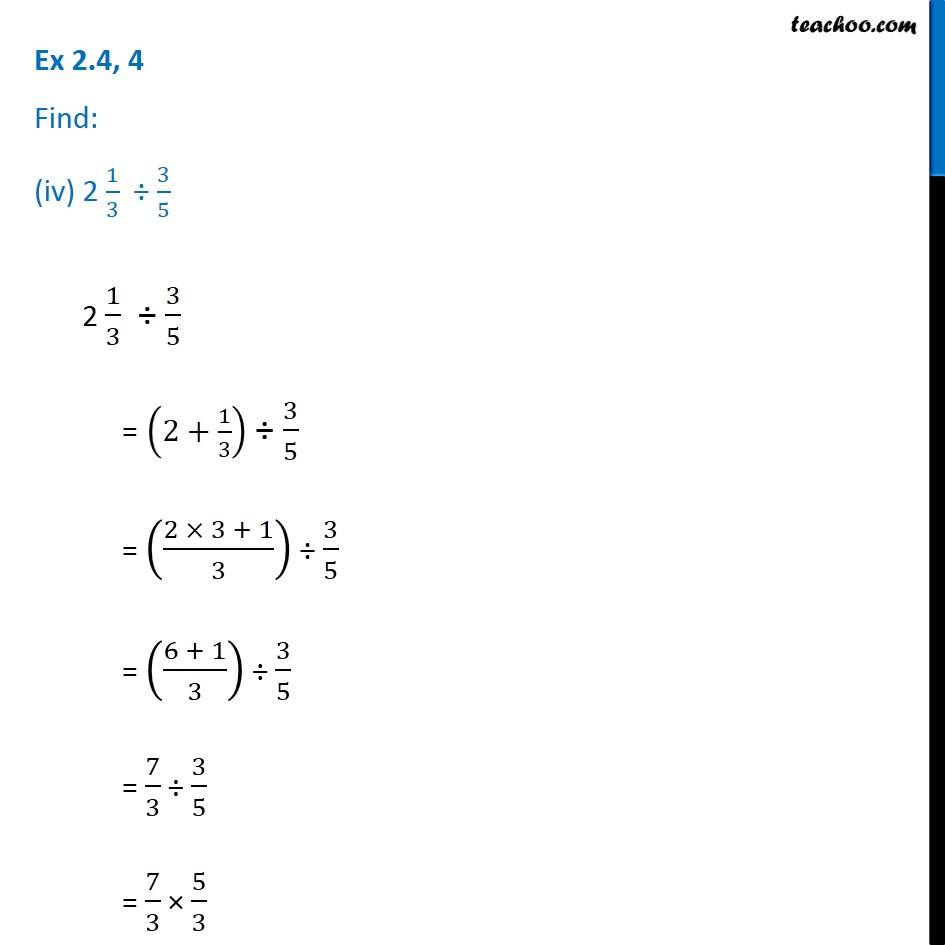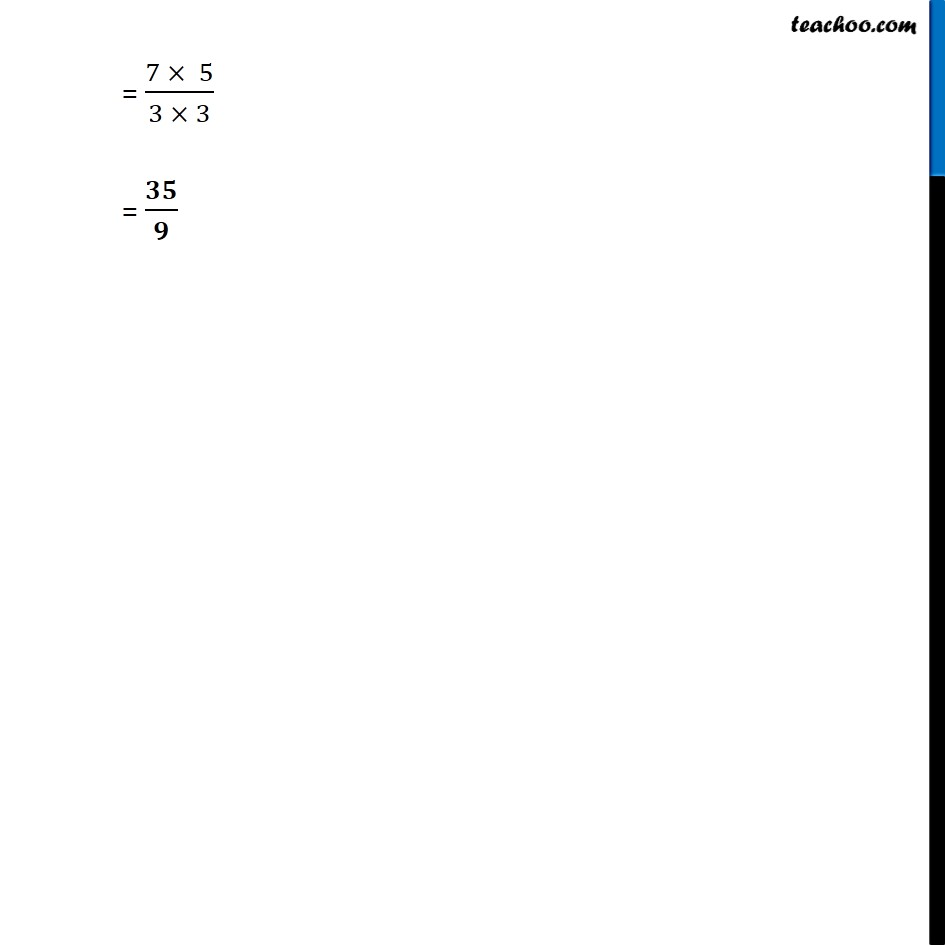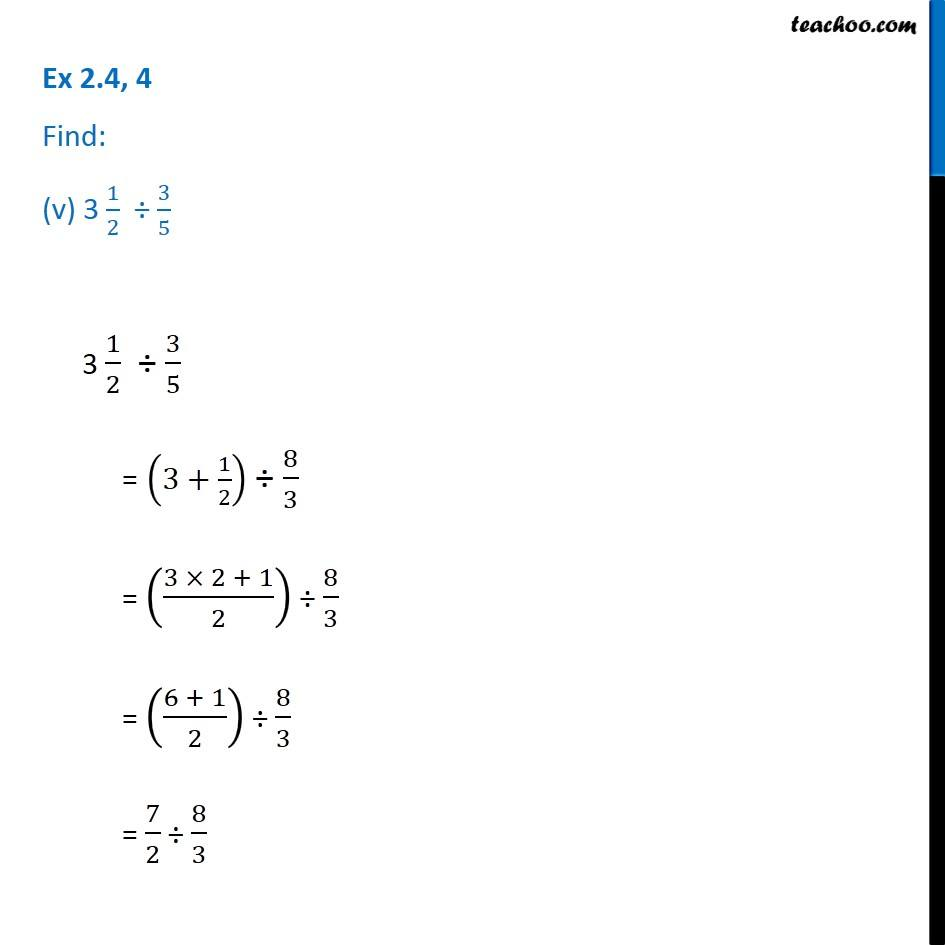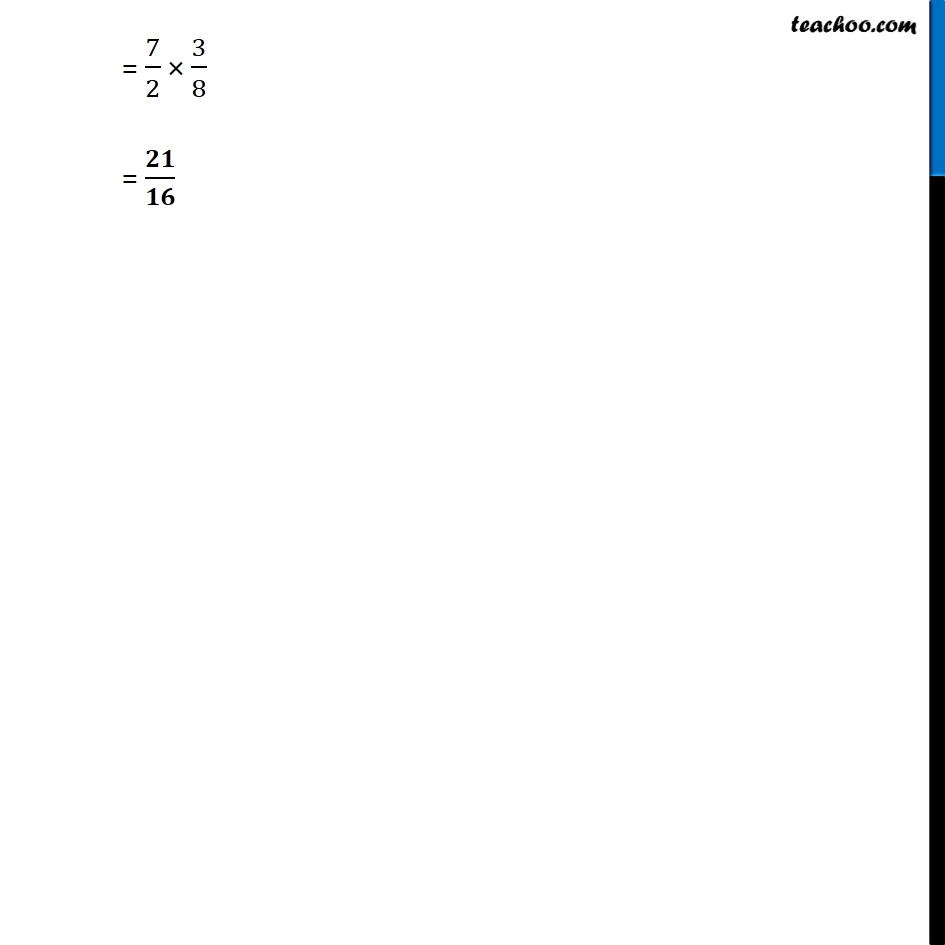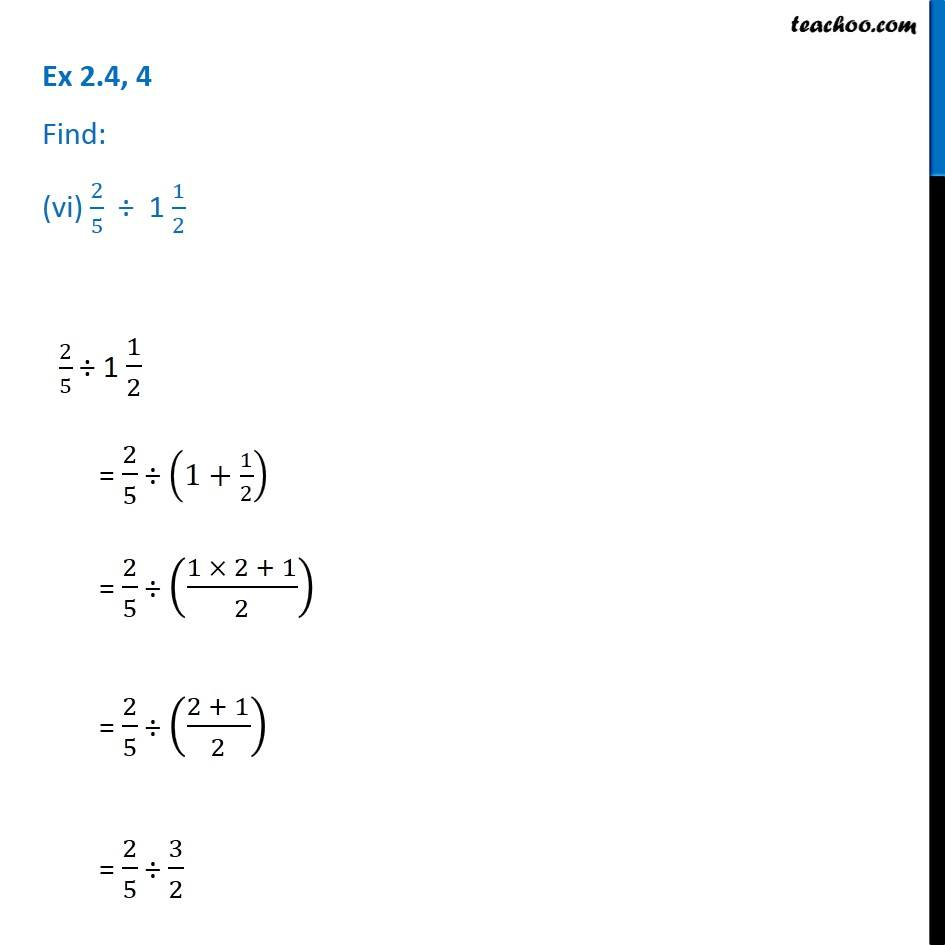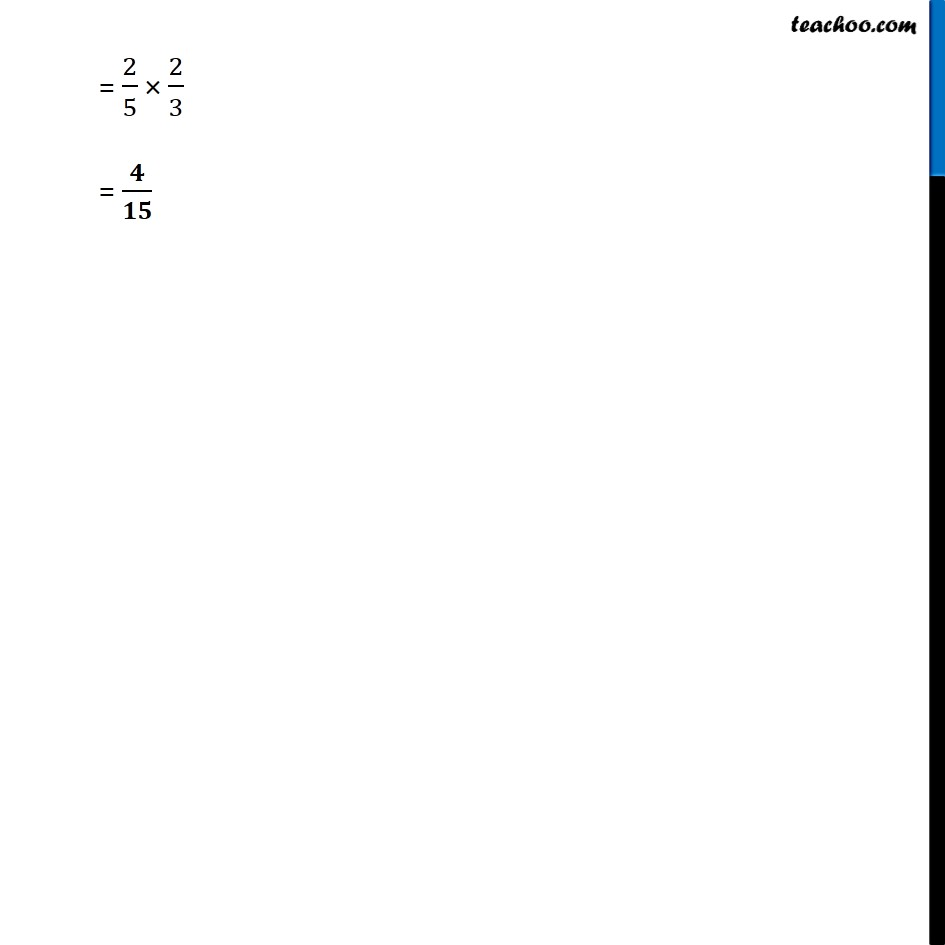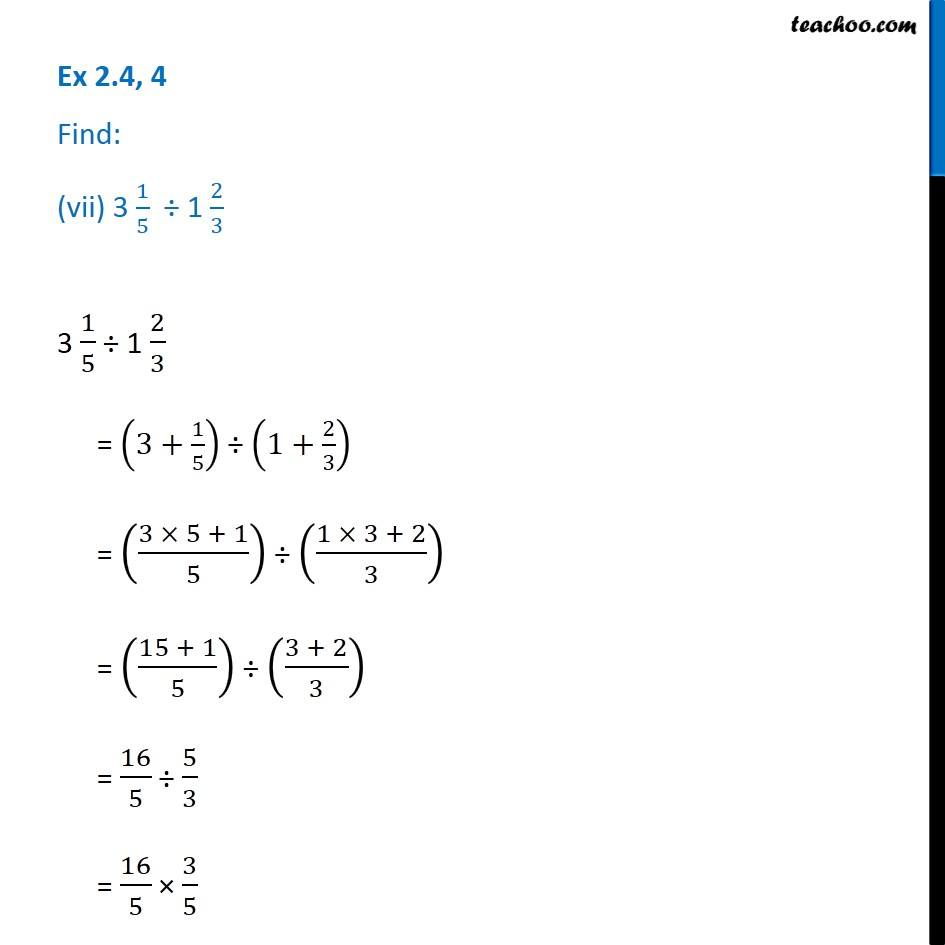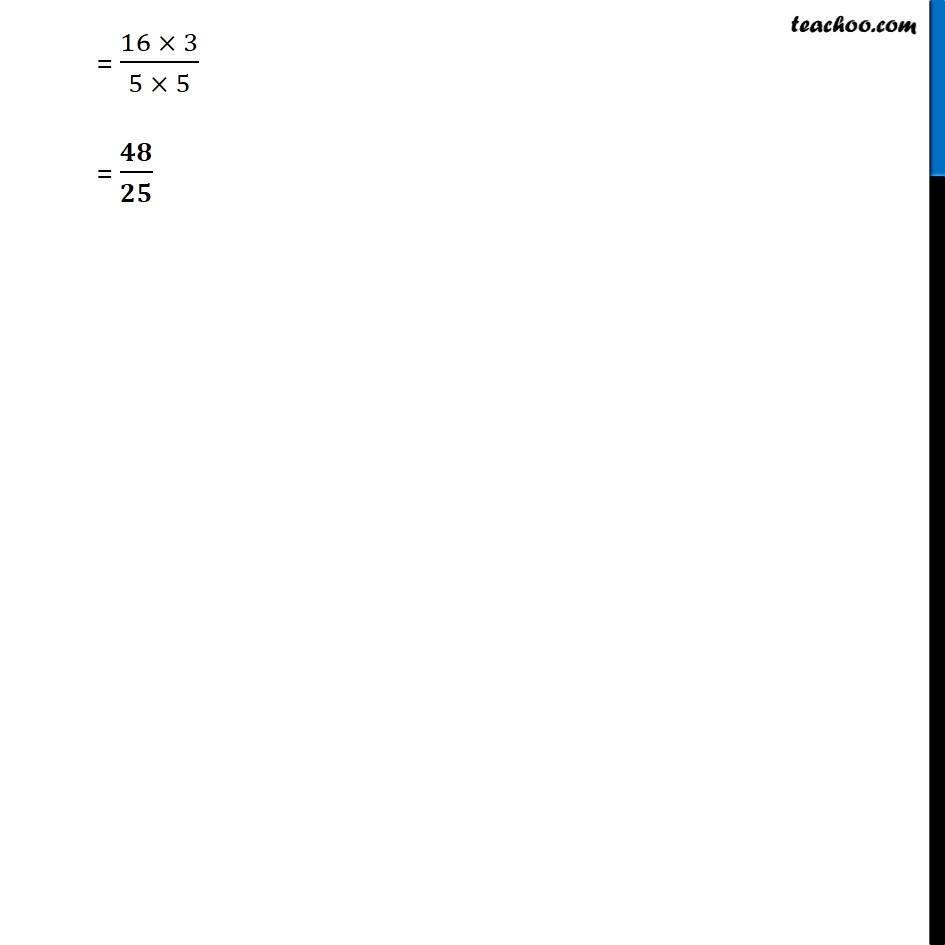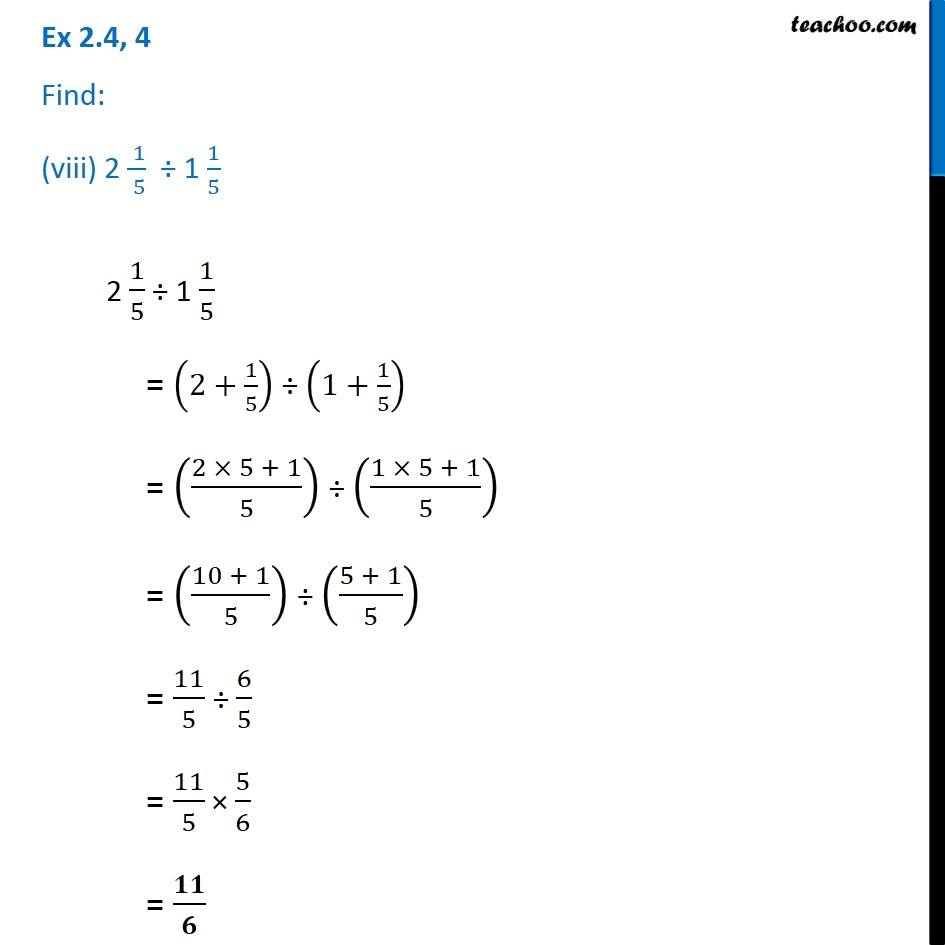1. Chapter 2 Class 7 Fractions and Decimals
2. Serial order wise
3. Ex 2.4

Transcript

Ex 2.4, 4 Find: (i) 2/5 ÷ 1/2 2/5 ÷ 1/2 = 2/5 × 2/1 = 𝟒/𝟓 Ex 2.4, 4 Find: (ii) 4/9 ÷ 2/3 4/9 ÷ 2/3 = 4/9 × 3/2 = (4 × 3)/(9 × 2) = (2 × 3)/9 = 𝟐/𝟑 Ex 2.4, 4 Find: (iii) 3/7 ÷ 8/7 3/7 ÷ 8/7 = 3/7 × 7/8 = 𝟑/𝟖 Ex 2.4, 4 Find: (iv) 2 1/3 ÷ 3/5 2 1/3 ÷ 3/5 = (2+1/3) ÷ 3/5 = ((2 × 3 + 1)/3) ÷ 3/5 = ((6 + 1)/3) ÷ 3/5 = 7/3 ÷ 3/5 = 7/3 × 5/3 = (7 × 5)/(3 × 3) = 𝟑𝟓/𝟗 Ex 2.4, 4 Find: (v) 3 1/2 ÷ 3/5 3 1/2 ÷ 3/5 = (3+1/2) ÷ 8/3 = ((3 × 2 + 1)/2) ÷ 8/3 = ((6 + 1)/2) ÷ 8/3 = 7/2 ÷ 8/3 = 7/2 × 3/8 = 𝟐𝟏/𝟏𝟔 Ex 2.4, 4 Find: (vi) 2/5 ÷ 1 1/2 2/5 ÷ 1 1/2 = 2/5 ÷ (1+1/2) = 2/5 ÷ ((1 × 2 + 1)/2) = 2/5 ÷ ((2 + 1)/2) = 2/5 ÷ 3/2 = 2/5 × 2/3 = 𝟒/𝟏𝟓 Ex 2.4, 4 Find: (vii) 3 1/5 ÷ 1 2/3 3 1/5 ÷ 1 2/3 = (3+1/5) ÷ (1+2/3) = ((3 × 5 + 1)/5) ÷ ((1 × 3 + 2)/3) = ((15 + 1)/5) ÷ ((3 + 2)/3) = 16/5 ÷ 5/3 = 16/5 × 3/5 = (16 × 3)/(5 × 5) = 𝟒𝟖/𝟐𝟓 Ex 2.4, 4 Find: (viii) 2 ( 1)/( 5) ÷ 1 1/5 2 1/5 ÷ 1 1/5 = (2+1/5) ÷ (1+1/5) = ((2 × 5 + 1)/5) ÷ ((1 × 5 + 1)/5) = ((10 + 1)/5) ÷ ((5 + 1)/5) = 11/5 ÷ 6/5 = 11/5 × 5/6 = 𝟏𝟏/𝟔

Ex 2.4